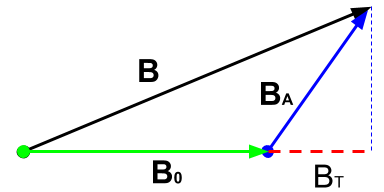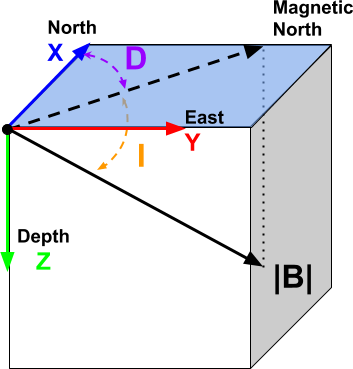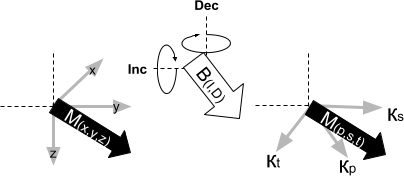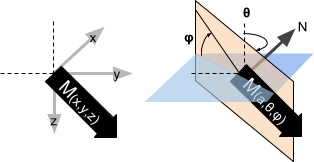# 2. Background theory¶

## 2.1. Introduction¶

Here we present the theoretical background of the magnetic problem, numerical examples, and details regarding the algorithms used by the MVI program library. This suite of algorithms, developed at the UBC-Geophysical Inversion Facility, is used to invert magnetic responses over a three-dimensional vector magnetization model. The manual is designed to help geophysicist who may be familiar with the magnetic experiment but not necessarily versed in the details of inverse theory.

Note

For more general information about the magnetic experiment, the reader is invited to visit the GPG site

## 2.2. Magnetic Data¶

Magnetic survey data are generally comprised of a set of total magnetic intensity (TMI) measurements acquired above the Earth’s surface; although borehole data are sometime collected. The observed magnetic datum $$b^{TMI}$$ can be written as:

$b^{TMI} = |\mathbf{B}_0 + \mathbf{B}_A|$

where both the Earth’s field $$\mathbf{B}_0$$ and the anomalous fields $$\mathbf{B}_A$$ from magnetized bodies are recorded. The goal of the magnetic inversion is to obtain information about the distribution of subsurface magnetization from the data. The assumption is usually made that the anomalous field is small compared to Earth’s field, thus the following is true:

(2.1)$\mathbf{B}_A \approx (\mathbf{B}_A \cdot \mathbf{\hat B}_0)\; \mathbf{\hat B}_0$

where $$\bf{\hat B}_0$$ is the unit vector in the direction of Earth’s field. The Total Magnetic Anomaly can therefore be defined as

${b^{TMA}} = b^{TMI} - |\mathbf{B}_0|\;,$## 2.3. Forward modelling¶

### 2.3.1. General formulation¶

The anomalous field produced by a distribution of magnetization $$\mathbf{M}$$ is given by the following integral equation, where the integrand is comprised of a dyadic Green’s function:

(2.2)$\mathbf{B}_A(\mathbf{r})=\frac{\mu_0}{4\pi}\int\limits_V \nabla \nabla \frac{1}{\left |\mathbf{r}-\mathbf{r}_0\right |}\cdot\mathbf{M} \; dv,$

where $$\mathbf{r}$$ is the observation location and $$V$$ represents the volume of magnetization at source locations $$\mathbf{r}_0$$. The above equation is valid for observation locations above the earth’s surface; i.e. outside the region of magnetization.

The total magnetization exhibited by a rock $$\mathbf{M}$$ is comprised of three components:

(2.3)$\mathbf{M} = \kappa \left( \mathbf{H}_0 + \mathbf{H}_s \right) + \mathbf{M}_r\;,$

where $$\boldsymbol{\kappa}$$ is the magnetic susceptibility of the rock, $$\mathbf{H_0}$$ is the Earth’s primary field, $$\mathbf{H_s}$$ represents any ambient secondary (self-demagnetizing) fields and $$\mathbf{M_r}$$ represents the contribution due to magnetic remanence. The total induced magnetization is given by $$\boldsymbol{\kappa (H_0 + H_s)}$$.

The magnetization of an object produces a magnetic flux. Outside the magnetized body, the magnetic flux density $$\mathbf{B}$$ and the magnetic field $$\mathbf{H}$$ are related by the magnetic permeability of free- space $$\mu_0$$ such that:

$\mathbf{H}=\mathbf{B} / \mu_0$

When the susceptibility is constant within a volume of source region, the Eq. (2.2) can be written in matrix form as:

(2.4)$\begin{split}\mathbf{B}_A=\mu_0\left( \begin{array}{ccc} T_{11} & T_{12} & T_{13} \\ T_{21} & T_{22} & T_{23}\\ T_{31} & T_{32} & T_{33} \end{array} \right)\mathbf{M} \equiv \mu_0\mathbf{T}\mathbf{M}.\end{split}$

The tensor $$\mathbf{T}_{ij}$$ is given by

(2.5)$\mathbf{T}_{ij}=\frac{1}{4\pi}\int\limits_V\frac{\partial}{\partial x_i}\frac{\partial}{\partial x_j}\frac{1}{\left |\mathbf{r}-\mathbf{r}_0\right |}dv, \mbox{ for }i=1,3 ; j=1,3,$

where $$x_1$$, $$x_2$$, and $$x_3$$ represent $$x-, y-$$, and $$z-$$directions, respectively. The expressions of $$\mathbf{T}_{ij}$$ for a cuboidal source volume can be found in [Bha64] and [Sha66]. Since $$\mathbf{T}$$ is symmetric and its trace is equal to $$-1$$ when the observation is inside the cell and is $$0$$ when the observation is outside the cell, only five independent elements need to be calculated.

Once $$\mathbf{T}$$ is formed, the magnetic anomaly $$\mathbf{B}_A$$ is easily obtained. Furthermore, its projection along any measurement direction is easily obtained by taking the inner product with the directional vector. The projection of the field $$\mathbf{B}_A$$ along different directions yields different anomalies commonly obtained in magnetic surveys. For instance, the vertical anomaly is simply $$B_{A_z}$$, the vertical component of $$\mathbf{B}_A$$, whereas the total field anomaly is, to first order, the projection of $$\mathbf{B}_A$$ onto the direction of the inducing field $$\mathbf{B}_0$$.

### 2.3.2. Numerical implementation of forward modelling¶Fig. 2.1 Right-hand coordinate system, z-axis positive down.

We use a right-handed coordinate system with z-axis pointing down. By equation (2.3), we divide the region of interest into a set of 3D prismatic cells and assume a constant magnetization within each cell from which we calculate the total anomalous field using equations (2.1) and (2.4). As input parameters within the data file, the coordinates, inclination and declination of the anomaly direction must be specified for each datum.

We can define the magnetization vector in terms of an effective susceptibility $$\boldsymbol \kappa_e$$ along the Cartesian directions such that

$\mathbf{M} = {H}_0 \boldsymbol \kappa_e$

and

$\begin{split}\boldsymbol \kappa_e = \left[ \begin{array}{c} \boldsymbol \kappa_x \\ \boldsymbol \kappa_y \\ \boldsymbol \kappa_z \end{array} \right]\end{split}$

Let the set of extracted anomaly data be $$\mathbf{d} = (d_1,d_2,...,d_N)^T$$ and the effective susceptibilities of cells in the model be $$\boldsymbol \kappa_e = (\kappa_{x_1},\kappa_{x_2},...,\kappa_{z_M})^T$$. The two are related by the forward matrix

(2.6)$\mathbf{d}=\mathbf{G}{\boldsymbol{\mathbf{m}}}.$

The matrix has elements $$g_{ij}$$ which quantify the contribution to the $$i^{th}$$ datum due to a unit susceptibility in the $$j^{th}$$ cell. The calculation involves the evaluation of equation (2.5) in a 3D rectangular domain defined by each cell. This operation can be done by MVIFWR if only the data is required, or by MVISEN if the forward matrix is stored on disk for the inversion. The $$G$$ matrix provides the forward mapping from the model to the data during the entire inverse process. We will discuss its efficient representation via the wavelet transform in a separate section.

## 2.4. Inversion methodology¶

The inverse problem is formulated as an optimization problem where a global objective function, $$\phi$$, is minimized subject to the constraints in equation (2.6). The global objective functions consists of two components: a model objective function, $$\phi_m$$, and a data misfit function, $$\phi_d$$, such that

(2.7)\begin{split}\begin{aligned} \min \phi = \phi_d+\beta\phi_m \\ \mbox{s. t. } \mathbf{m}^l\leq \mathbf{m} \leq \mathbf{m}^u, \nonumber\end{aligned}\end{split}

where $$\beta$$ is a trade off parameter that controls the relative importance of the model smoothness through the model objective function and data misfit function. When the standard deviations of data errors are known, the acceptable misfit is given by the expected value $$\phi_d$$ and we will search for the value of $$\beta$$ via an L-curve criterion [Han00] that produces the expected misfit. Otherwise, a user-defined $$\beta$$ value is used. Bounds are imposed through the projected gradient method so that the recovered model lies between imposed lower ($$\mathbf{m}^l$$) and upper ($$\mathbf{m}^u$$) bounds.

In discrete matrix form, the objective function in (2.7) can be written as

$\phi = \phi_d + \beta \phi_m = \| \mathbf{W}_d (\mathbb{F}(\mathbf{m}) - \mathbf{d}^{obs})\|_2^2 +\beta \sum_{i = s,x,y,z} {\|\mathbf{W_i}(\mathbf{m-m_{ref}})\|}^2_2 \;,$

where $$\mathbf{W}_i$$ are functions measuring the deviation of the model $$\mathbf{m}$$ from a reference $$\mathbf{m_{ref}}$$ or the roughness measured along three orthogonal directions. The following sections provide additional details about the misfit and the regularization function.

## 2.5. Misfit function $$\phi_d$$¶

The first term in (2.7) defines a measure of how well the observed data are reproduced by a model $$\mathbf{m}$$. Here we use the $$l_2$$-norm measure

(2.8)\begin{aligned} \phi_d = \left\| \mathbf{W}_d(\mathbb{F}(\mathbf{m})-\mathbf{d})\right\|^2.\end{aligned}

For the work here, we assume that the contaminating noise on the data is independent and Gaussian with zero mean. Specifying $$\mathbf{W}_d$$ to be a diagonal matrix whose $$i^{th}$$ element is $$1/\sigma_i$$, where $$\sigma_i$$ is the standard deviation of the $$i^{th}$$ datum, makes $$\phi_d$$ a chi-squared distribution with $$N$$ degrees of freedom. The optimal data misfit for data contaminated with independent, Gaussian noise has an expected value of $$E[\chi^2]=N$$, thus providing a target misfit for the inversion. We now have the components to solve the inversion as defined in equation (2.7).

To solve the optimization problem when constraints are imposed we use the projected gradients method [CM87][Vog02]. This technique forces the gradient in the Krylov sub-space minimization (in other words a step during the conjugate gradient process) to zero if the proposed step would make a model parameter exceed the bound constraints. The result is a model that reaches the bounds, but does not exceed them.

### 2.5.1. Sensitivities¶

A solution to (2.7) is found by the second order Gauss-Newton method, such that a model update is calculated by iteratively solving

(2.9)$\begin{split}\frac{\partial \phi(\mathbf{m})}{\partial \mathbf{m}} = \mathbf{J^T W_\text{d}^T W_\text{d}} \left[ \mathbb{F}(\mathbf{m}) -\mathbf{d}^{obs} \right]+ \beta \mathbf{W^T} \mathbf{W} ( \mathbf{m} - \mathbf{m_{ref}}) \\\end{split}$

where $$\mathbf{J}$$, also known as the sensitivity matrix, holds the derivatives of the forward operation with respect to the model

$\mathbf{J} = \frac{\partial \mathbb{F}(\mathbf{m})}{\partial \mathbf{m}}$

The first question that arises during the inversion of magnetic data concerns the definition of the “model”. The MVI program allows for the inversion of a magnetization vector defined in either Cartesian or Spherical coordinate systems [Lel09]. We define both systems below.

#### 2.5.1.1. Cartesian (PST)¶

The first choice is to define a model $$\mathbf{m}$$ in terms of effective magnetic susceptibility $$\boldsymbol \kappa_e$$ along a rotated coordinate system such that one of the components is aligned with the inducing field $$\mathbf{H}_0$$. Thus

$\begin{split}\mathbf{M} = |{H}_0| \left[ \begin{array}{c} \boldsymbol \kappa_P \\ \boldsymbol \kappa_S \\ \boldsymbol \kappa_T \end{array} \right]\\ \mathbf{m}_{PST} = \Omega_\phi \Omega_\theta \boldsymbol \kappa_{xyz}\end{split}$

where p (primary), s (secondary) and t (tertiary) defines an orthogonal system that describes the magnetization vector in 3D. The matrices $$\Omega_\theta$$ and $$\Omega_\phi$$ define the rotation around the z-axis and y-axis respectively so that the x-axis points along the inducing field direction.Fig. 2.2 Cartesian PST rotated coordinate system.

The sensitivity matrix $$\mathbf{J}$$ simplifies to

$\begin{split}\mathbf{J} = \frac{\partial \mathbb{F}(\mathbf{m})}{\partial \mathbf{m}} = \mathbf{\tilde G} \\ \mathbf{\tilde G} = \mathbf{G} \Omega_\phi \Omega_\theta\end{split}$

The main advantage of this formulation is that the inversion remains linear. The drawback is that both the direction and the magnitude of magnetization are coupled in the vector components, which makes it harder to impose constraints on the magnetization vector through sparsity and/or petrophysical constraints.

#### 2.5.1.2. Spherical (ATP)¶

As an alternative to the Cartesian formulation, the magnetization vector can be expressed in terms of an amplitude ($$\alpha$$) and two orientation angles ($$\theta,\;\phi$$) (ATP).

$\begin{split}\mathbf{m}_{ATP} = \left[ \begin{array}{c} \mathbf{a} \\ \boldsymbol \theta \\ \boldsymbol \phi \end{array} \right]\end{split}$
(2.10)$\begin{split}x =& \alpha \; cos(\theta)\;cos(\phi) \\ y = & \alpha \; cos(\theta)\;sin(\phi) \\ z = & \alpha \; sin(\theta)\end{split}$Fig. 2.3 Spherical (ATP) coordinate system.

The sensitivity matrix becomes non-linear due to the trigonometric transformation such that

$\mathbf{J} = \frac{\partial \mathbb{F}(\mathbf{m})}{\partial \mathbf{m}} = \mathbf{G}\;\mathbf{S}$

where the matrix $$\mathbf{S}$$ holds the partial derivatives of (2.10)

$\begin{split}\mathbf{S} = \begin{bmatrix} \cos{\phi}\cos{\theta} & -\alpha\sin{\phi}\cos{\theta} & -\alpha\cos{\phi}\sin{\theta} \\ \cos{\phi}\sin{\theta} & -\alpha\sin{\phi}\sin{\theta} & \alpha\cos{\phi}\cos{\theta} \\ \sin{\phi} & \alpha\cos{\phi} & 0 \end{bmatrix}\end{split}$

Up until recently, solving the spherical formulation had proven to be prohibitively difficult. Issues regarding the convergence of the non-linear problem have now been addressed through an automated sensitivity re-weighting strategy.

Solving for model parameters in spherical coordinates comes with the increased flexibility, as the user constrains the amplitude and orientation independently. The reader is encouraged to visit the examples section.

### 2.5.2. Regularization¶

We next discuss the construction of a model objective function which, when minimized, produces a model that is geophysically interpretable. This function gives the flexibility to incorporate as little or as much information as possible. At minimum, it drives the solution towards a reference model $$\mathbf{m}_0$$ and requires that the model be relatively smooth in the three spatial directions. Let the model objective function expressed as

(2.11)$\begin{split}\phi_m(\mathbf{m}) = \alpha_s\int\limits_V w_s w(\mathbf{r})\left|\mathbf{m}(\mathbf{r})-{\mathbf{m_{ref}}}\right|^{p_s} dv \;+\\ \sum_{i=x,y,z} \alpha_i\int\limits_V w_i w(\mathbf{r})\left|\frac{\partial [\mathbf{m}(\mathbf{r})-{\mathbf{m_{ref}}}]}{\partial i}\right|^{p_i} dv \\ \nonumber\end{split}$

where the functions $$w_s$$, $$w_x$$, $$w_y$$ and $$w_z$$ are user defined weights, , while $$\alpha_s$$, $$\alpha_x$$, $$\alpha_y$$ and $$\alpha_z$$ are coefficients which affect the relative importance between the penalty functions. Each function can use different $$l_p$$-norm measures to enforce sparsity on model or its gradients. The reference model $$\mathbf{m_{ref}}$$ can be included optionally in the gradient penalty through the SMOOTH_MOD_DIF option. The generalized sensitivity weighting function $$w(\mathbf{r})$$ counteract the geometrical decay of the sensitivities with respect to the distances from the observation locations. The details of the sensitivity weighting function will be discussed in the next section.

The $$l_p$$-norm is approximated by a Scaled-IRLS procedure such that for some general model function $$f(m)$$ (model or its gradients)

$\int | f(m) |^p dV \approx \int \frac{f(m)^2}{(f(m^{(k-1)})^2 + \epsilon^2)^{1-p/2}} dV$

where $$k$$ stands for the iteration step. Numerically, the model objective function in equation Eq. (2.11) is discretized onto the mesh using a finite difference approximation. This yields:

(2.12)$\phi_m({\mathbf{m}}) = \alpha_s \| \mathbf{W}_s \mathbf{R_s} ({\mathbf{m}}-{\mathbf{m_{ref}}})\|_2^2 + \sum_{i=x,y,z} \alpha_i \| \mathbf{W}_i \mathbf{R_i} \mathbf{G}_i (\mathbf{m}-\mathbf{m_{ref}}),$

where $$\mathbf{m}$$ and $$\mathbf{m}_0$$ are vectors of length $$3M$$ representing the recovered and reference models, respectively. The individual matrices $$\mathbf{W}_s$$, $$\mathbf{W}_x$$, $$\mathbf{W}_y$$, and $$\mathbf{W}_z$$ contain user-defined weights as well as the sensitivity weighting functions $$w(\mathbf{r})$$. The gradient matrices $$\mathbf{G}_x$$, $$\mathbf{G}_y$$ and $$\mathbf{G}_z$$ are finite difference operators measuring the change in model values.

The diagonal matrices $$\mathbf{R}_s$$, $$\mathbf{R}_x$$, $$\mathbf{R}_y$$ and $$\mathbf{R}_z$$ contain sparsity weights applied on the model and its gradients:

$\mathbf{R} = diag(\left[\gamma (f(\mathbf{m}^{(k-1)})^2 + \epsilon^2)^{p/2-1} \right]^{1/2})$

where $$\gamma$$ is a normalization factor. More details about the sparse inversion can be found here By default (and for PST formulation) the inversion uses the $$l_2$$-norm penalty, in which case the $$\mathbf{R}$$ matrices reduce to the indentity matrix. Note that $$p \in [0,2]$$ can be defined on a cell-by-cell basis.

Important

Change from previous versions - The difference operators $$\mathbf{G_i}$$ are now unitless, removing the need to alter scaling between the smallness and smoothness terms. By default, $$\alpha_s=\alpha_x=\alpha_y=\alpha_z = 1$$

## 2.6. Sensitivity Weighting¶

It is a well-known fact that static magnetic data have no inherent depth resolution. Thus when an inversion is performed which minimizes $$\int m(\mathbf{r})^2 dv$$ subject to fitting the data, the constructed susceptibility is concentrated close to the observation locations. This is a direct manifestation of the kernel’s decay with respect to the distance between the cell and observation locations. Because of the rapidly diminishing amplitude, the kernels of magnetic data are not sufficient to generate a function that possess significant structure at locations that are far away from observations.

Moreover, the trigonometric transformation associated with the spherical formulation introduces rapid changes in the sensitivity function, which affects the convergence of the algorithm.

In order to overcome these issues, we opt for an iterative re-weighting of the regularization to adjust the relative influence of the misfit and regularization functions. While previous version of the MAG3D and MVI made use of a depth or distance weighting, in this version we calculate the weights directly from the sensitivity matrix. We define the sensitivity weights as follow:

(2.13)$\begin{split}\mathbf{W_r} &= diag \left( {\left[{\mathbf{\hat w_r}}\right]}^{1/2}\right)\\ \mathbf{\hat w_{r}} &= \frac{\mathbf{ w_{r}}}{max(\mathbf{ w_{r}})}\\ w_{r_j} &= {\left[\sum_{i=1}^{nD}{J^{(k)}_{ij}}^2 + \delta \right]}^{1/2}\;,\end{split}$

where the superscript $$(k)$$ is an iteration index and $$\delta$$ is a small number added to avoid singularity.

## 2.7. Wavelet Compression of Sensitivity Matrix¶

The two major obstacles to the solution of a large-scale magnetic inversion problem are the large amount of memory required for storing the sensitivity matrix and the CPU time required for the application of the sensitivity matrix to model vectors. This program library overcomes these difficulties by forming a sparse representation of the sensitivity matrix using a wavelet transform based on compactly supported, orthonormal wavelets. For more details, the users are referred to [LO03][LO10]. Here, we give a brief description of the method necessary for the use of the MVI library.

Each row of the sensitivity matrix in a 3D magnetic inversion can be treated as a 3D image and a 3D wavelet transform can be applied to it. By the properties of the wavelet transform, most transform coefficients are nearly or identically zero. When coefficients of small magnitude are discarded (the process of thresholding), the remaining coefficients still contain much of the necessary information to reconstruct the sensitivity accurately. These retained coefficients form a sparse representation of the sensitivity in the wavelet domain. The need to store only these large coefficients means that the memory requirement is reduced. Furthermore, the multiplication of the sensitivity with a vector can be carried out by a sparse multiplication in the wavelet domain. This greatly reduces the CPU time. Since the matrix-vector multiplication constitutes the core computation of the inversion, the CPU time for the inverse solution is reduced accordingly. The use of this approach increases the size of solvable problems by nearly two orders of magnitude.

Let $$\mathbf{G}$$ be the sensitivity matrix and $$\mathcal{W}$$ be the symbolic matrix-representation of the 3D wavelet transform. Applying transform to each row of $$\mathbf{G}$$, and forming a new matrix consisting of rows of transformed sensitivity, is equivalent to the following operation:

(2.14)$\widetilde{\mathbf{G}}=\mathbf{G}\mathcal{W}^T,$

where $$\widetilde{\mathbf{G}}$$ is the transformed matrix. The thresholding is applied to individual rows of $$\mathbf{G}$$ by the following rule to form the sparse representation $$\widetilde{\mathbf{G}}^S$$,

(2.15)$\begin{split}\widetilde{g}_{ij}^{s}=\begin{cases} \widetilde{g}_{ij} & \mbox{if } \left|\widetilde{g}_{ij}\right| \geq \delta _i \\ 0 & \mbox{if } \left|\widetilde{g}_{ij}\right| < \delta _i \end{cases}, ~~ i=1,\ldots,N,\end{split}$

where $$\delta _i$$ is the threshold level, and $$\widetilde{g}_{ij}$$ and $$\widetilde{g}_{ij}^{s}$$ are the elements of $$\widetilde{\mathbf{G}}$$ and $$\widetilde{\mathbf{G}}^S$$, respectively. The threshold levels $$\delta _i$$ are determined according to the allowable error of the reconstructed sensitivity, which is measured by the ratio of norm of the error in each row to the norm of that row, $$r_i(\delta_i)$$. It can be evaluated directly in the wavelet domain by the following expression:

(2.16)$r_i(\delta_i)=\sqrt{\frac{\underset{\left | {\widetilde{g}_{ij}} \right| <\delta_i}\sum{\widetilde{g}_{ij}}^2}{\underset{j}\sum{\widetilde{g}_{ij}^2}}}, ~~i=1,\ldots,N,$

Here the numerator is the norm of the discarded coefficients and the denominator is the norm of all coefficients. The threshold level $$\delta_{i_0}$$ is calculated on a representative row, $$i_0$$. This threshold is then used to define a relative threshold $$\epsilon =\delta_{i_{o}}/ \underset{j}{\max}\left | {\widetilde{g}_{ij}} \right |$$. The absolute threshold level for each row is obtained by

(2.17)$\delta_i = \epsilon \underset{j}{\max}\left | {\widetilde{g}_{ij}} \right|, ~~i=1,\ldots,N.$

The program that implements this compression procedure is MVISEN. For experienced users, the program allows the direct input of the relative threshold level. However it is recommended newer users let the program determine the optimal compression accuracy.